# Bonding Position

Yet another related dimension of symmetry, when you combine the terminating digit symmetries described above, capturing three (3) rotations of 120 around the sieve in their actual sequences, you produce the ultimate combinatorial symmetry

``````---+-----+-----
1 | {1} |{11}
---+-----+-----
2 | 12  | 32
---+-----+-----
3 | 33  |{50}
---+-----+-----
4 | 51  |{86}
---+-----+-----
5 | 87  | 108
---+-----+-----
6 |{109}| 120
---+-----+-----
``````

In quantum mechanics, Schrödinger's cat is a thought experiment that illustrates a paradox of quantum superposition.

In the thought experiment, a hypothetical cat may be considered simultaneously both alive and dead, while it is unobserved in a closed box, as a result of its fate being linked to a random subatomic event that may or may not occur (Wikipedia).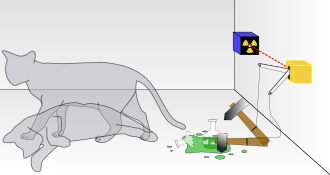2x10 + 9 = 20 + 9 = 29

``````-----+-----+-----+-----+-----+
1' |  1  | {2} |  3  |  4  | 4¤
+-----+-----+-----+-----+
2' |  5  |  6  |  7  |  8  | 4¤
+-----+-----+-----+-----+
3' |  9  |{10} |  2¤ (M dan F)
+-----+-----+-----+
4' | 11  | 12  | 13  | 3¤  <----------- d(11) = d(17+12)= d(29)
+-----+-----+-----+-----+
5' | 14  | 15  | 16  | 17  | 4¤
+-----+-----+-----+-----+
6' | 18  | 19  |{20} | 3¤
-----+-----+-----+-----+-----+-----+-----+-----+-----+-----+
∑  | 21  | 22  | 23  | 24  |{25} | 26  | 27  | 28  | 29  | 9¤
-----+-----+-----+-----+-----+-----+-----+-----+-----+-----+
|----------------------- {9'} ------------------------|
``````

Primzahlentechnologie Einleitung. Wie Geht Primzahlen Technologie. MEC 30 - Mathematical Elementary Cell 30: Die Theorie der Gravitation ist die Mathematik des Universums and MEC30.2 Method for factorization

Measuring with your limb scale is a measurement that does not intervene in the system, so also possible in the idle state of the system. Thus, the MEC 30 results in a measurement method for the energy flow in a system in which the route plan or flow plan of the energy is obtained (German Patent DE102011101032 and DE102014016656).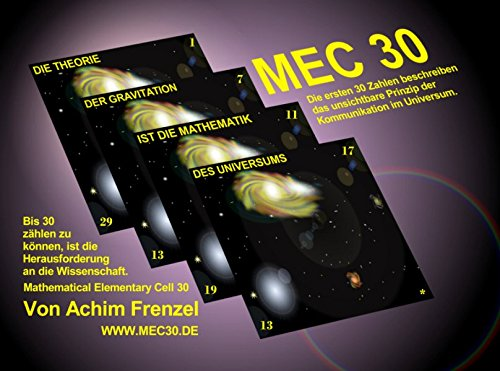The Prime Spiral Sieve possesses remarkable structural and numeric symmetries. For starters, the intervals between the prime roots (and every subsequent row or rotation of the sieve) are perfectly balanced, with a period eight (8) difference sequence of: {6, 4, 2, 4, 2, 4, 6, 2} (Primesdemystified).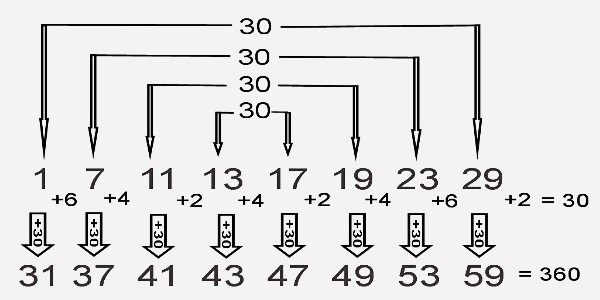## Symmetry Mirroring

Speaking of the Fibonacci number sequence, there is symmetry mirroring the above in the relationship between the terminating digits of Fibonacci numbers and their index numbers equating to members of the array populating the Prime Spiral Sieve.

The 77 is equal to the sum of the first 8 primes. The square of 77 is 5929, the concatenation of two primes, 59 and 29. The concatenation of all palindromes from one up to 77 is prime (Prime Curios! ).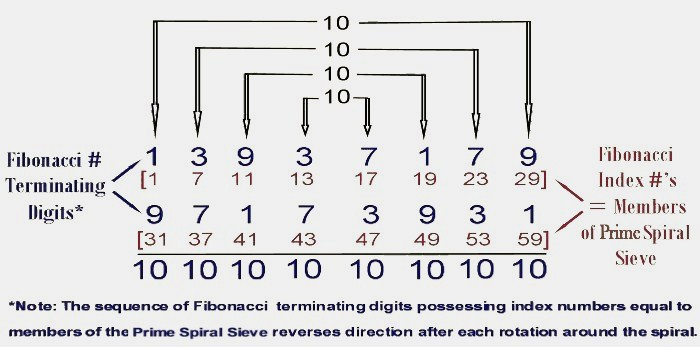Prime numbers that end with "77" occur more often than any other 2-digit ending among the first one million primes. The sum of the proper divisors of 77 equals 19 and the sum of primes up to 19 equals 77. Does this ever happen again?

``````  Tabulate Prime by Power of 10
loop(10) = π(10)-π(1) = 4-0 = 4
loop(100) = π(100)-π(10)-1th = 25-4-2 = 19
loop(1000) = π(1000) - π(100) - 10th = 168-25-29 = 114

-----------------------+----+----+----+----+----+----+----+----+----+-----
True Prime Pairs Δ    |  1 |  2 |  3 |  4 |  5 |  6 |  7 |  8 |  9 | Sum
=======================+====+====+====+====+====+====+====+====+====+=====
19 → π(10)            |  2 |  3 |  5 |  7 |  - |  - |  - |  - |  - | 4th  4 x Root
-----------------------+----+----+----+----+----+----+----+----+----+-----
17 → π(20)            | 11 | 13 | 17 | 19 |  - |  - |  - |  - |  - | 8th  4 x Twin
-----------------------+----+----+----+----+----+----+----+----+----+-----
13 → π(30) → 12 (Δ1)  | 23 | 29 |  - |  - |  - |  - |  - |  - |  - |10th
=======================+====+====+====+====+====+====+====+====+====+===== 1st Twin
11 → π(42)            | 31 | 37 | 41 |  - |  - |  - |  - |  - |  - |13th
-----------------------+----+----+----+----+----+----+----+----+----+----- 2nd Twin
7 → π(60) → 19 (Δ12) | 43 | 47 | 53 | 59 |  - |  - |  - |  - |  - |17th
-----------------------+----+----+----+----+----+----+----+----+----+----- 3rd Twin
5 → π(72) → 18 (Δ13) | 61 | 67 | 71 |  - |  - |  - |  - |  - |  - |20th
=======================+====+====+====+====+====+====+====+====+====+===== 4th Twin
3,2 → 18+13+12 → 43  | 73 | 79 | 83 | 89 | 97 | 101| 103| 107| 109|29th
=======================+====+====+====+====+====+====+====+====+====+=====
Δ                                                            Δ
12+13+(18+18)+13+12   ← 36th-Δ1=151-1=150=100+2x(13+12)   ←   30th = 113 = 114-1
``````

The individual bases, depending on their sizes and spatial extents and masses, are assigned unequivocal values, situated within the vibration heights derived from (I), to define a measure from which the sequence can be counted, read or imaged.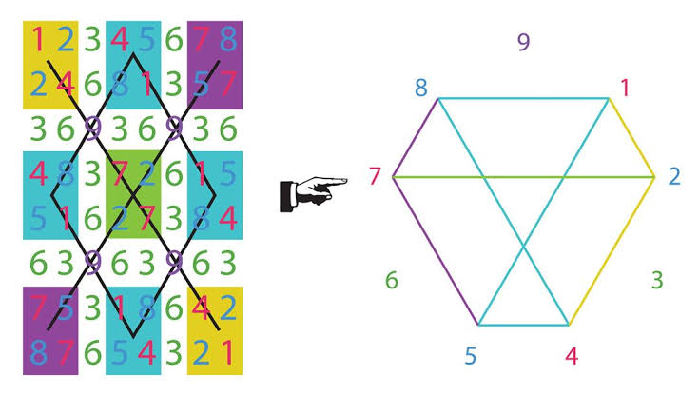``````layer | node | sub |    i     |   f
------+------+-----+----------+-----+-----+-----+                                    ---
|      |     |    1,2:1 |   1 |  30 |  40 | 71 (2,3) ‹-------------------       |
|      |  1  +----------+-----+-----+-----+                              |      |
|  1   |     |        2 |                                                |      5¨  encapsulation
|      |-----+----------+            -----------------------------       |      |
|      |     |        3 |           |                             |      |      |
1   +------+  2  +----------+----       |       LAGGING SCHEME        |      |     ---
|      |     |        4 |           |    (Exponentiation Zone)    |      |      |
|      +-----+----------+           |                             |      |      |
|  2   |     |        5 |           ------------------------------       |      7¨  abstraction
289   |      |  3  +----------+                                                |      |
|     |      |     |        6 |  ‹---------------------------- Φ               | {6®} |
------+------+-----+----------+-----+-----                                     |     ---
|      |     |     11:7 |   5 |   9 |  14 (20) --------› ¤               |      |
|      |  4  +----------+-----+-----+-----+                              |      |
|  3   |     |     12:8 |   9 |  60 |  40 | 109 (26) «------------       |     11¨  polymorphism
|      +-----+----------+-----+-----+-----+                       |      |      |
|      |     |     13:9 |   9 |  60 |  69 (27) «-- Δ19 (Rep Fork) | {2®} |      |
2   +------|  5  +----------+-----+-----+-----+                       |      |     ---
|      |     |    14:19 |   9 |  60 |  40 | 109 (28) -------------       |      |
|      |-----+----------+-----+-----+-----+                              |      |
|  4   |     | 15,18:11 |   1 |  30 |  40 | 71 (29,30,31,32) ------------      13¨  inheritance
329   |      |  6  +----------+-----+-----+-----+                                     |
|     |      |     |    19:12 |  10 |  60 | {70} (36) -------› Φ                      |
------+------+-----+----------+-----+-----+                                          ---
|      |     |    20:13 |  90 |  90 (38) ‹-------------- ¤                      |
|      |  7  +----------+-----+                                                 |
|  5   |     |       14 |            -----------------------------             17¨  class
|      |-----+----------+           |                             |             |
|      |     |       15 |           |       LEADING SCHEME        |             |
3   +------+  8  +----------+-----      |    (Multiplication Zone)    |            ---
|      |     |       16 |           |                             |             |
|      |-----+----------+-----+      -----------------------------              |
|  6   |     |    28:17 | 100 |                                                19¨  object
168   |      |  9  +----------+-----+                                                 |
|     |      |     |    29:18 | 50  | 50(68) ---------> Δ18                           |
------|------|-----+----------+-----+                                                ---
``````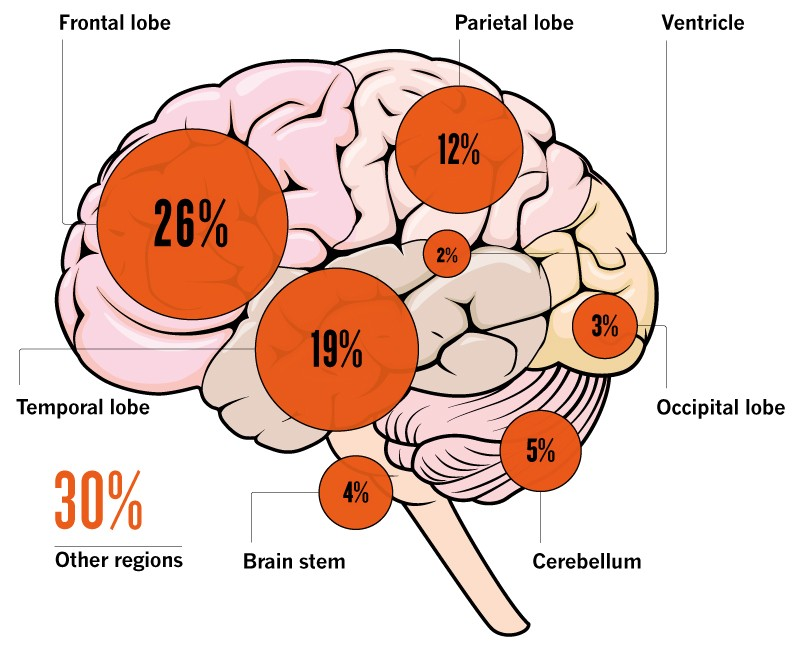## Molecular Interactions

By the nature this behaviour can be observed from the molecular interactions of water. Water is intrinsically self-complementary on molecular interactions. In liquid or solid water, engage in ideal hydrogen bonding. Six (6) times of the angle 109 occupied as the most while the angle of 114 and 104 are exist only once. So the one in charge here is clearly the 29th prime identity.

109 = 29th prime = (10th)th prime = ((114-104)th)th prime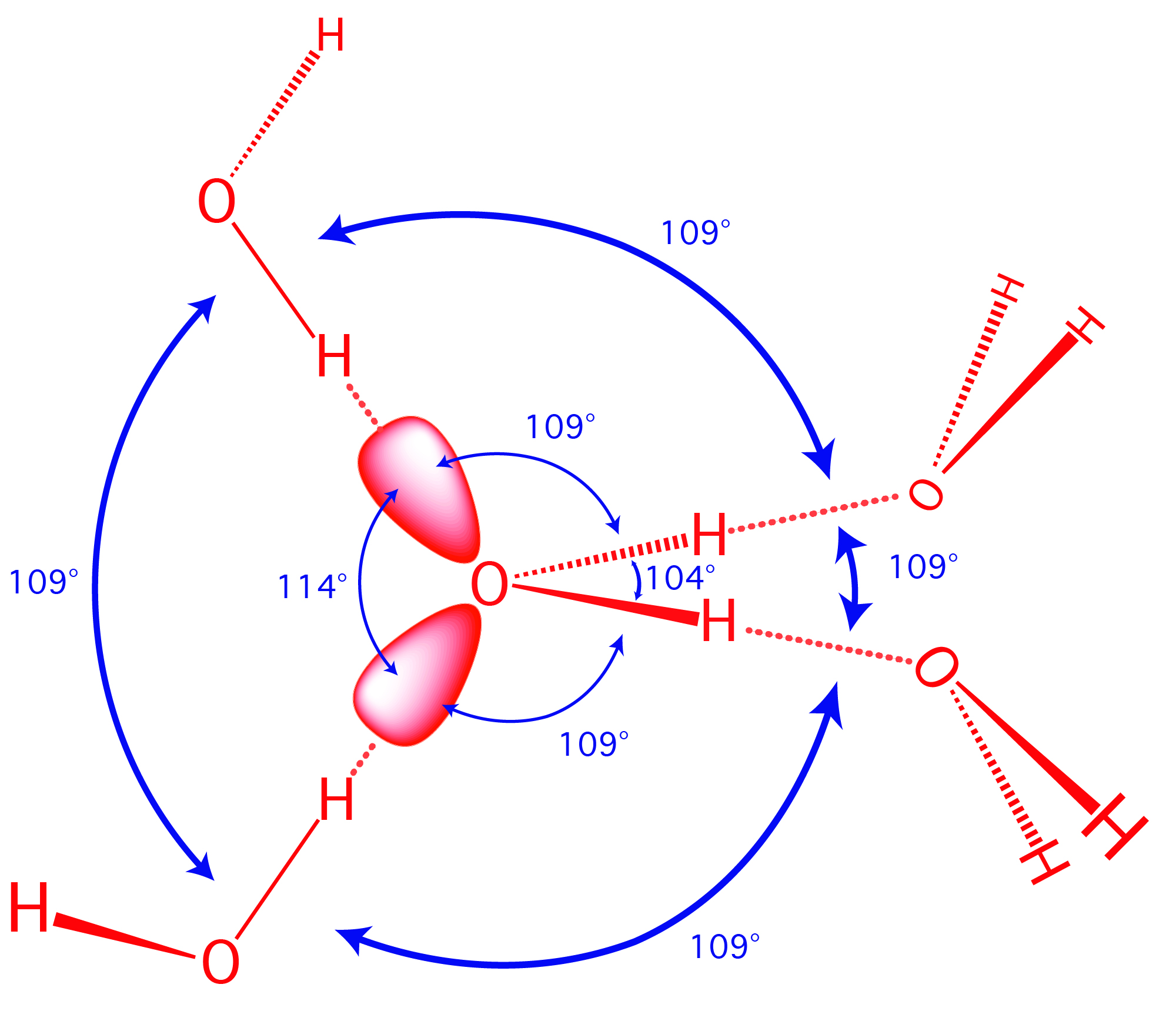Let's assume that it is done using a material that stretches and then pops back when the stretching force goes away. It is pound for pound stronger than steel. So all of these steps are similar kind with the way a spider started to build its web.

Every web begins with a single thread, which forms the basis of the rest of the structure. To establish this bridge, the spider climbs to a suitable starting point (up a tree branch, for example) and releases a length of thread into the wind. With any luck, the free end of the thread will catch onto another branch (howstuffworks.com).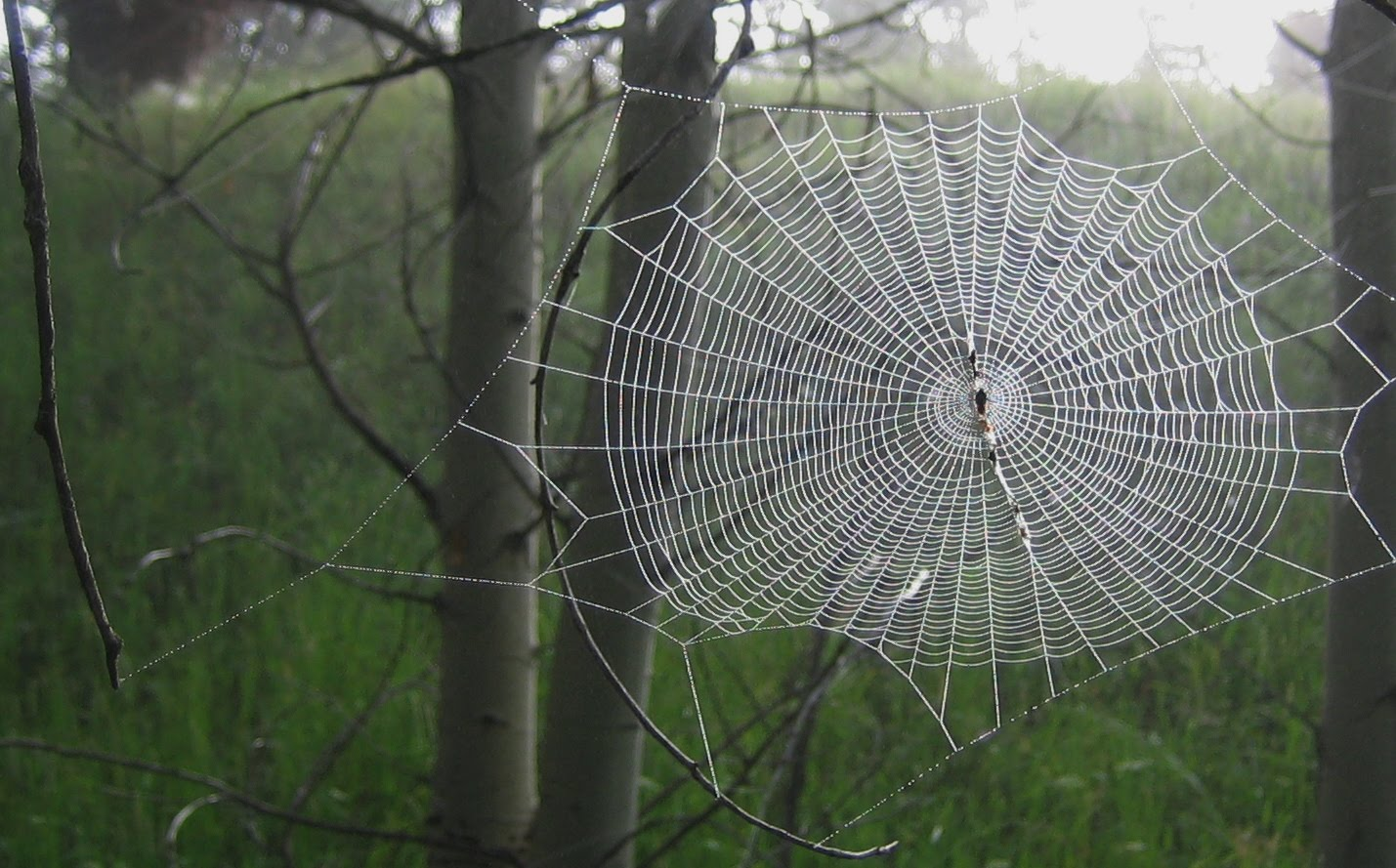This 29 turns the finiteness position of 15 as the middle zero axis of the MEC30. So the next steps will start exactly with the same story as we have explained from the beginning.

MEC 30 claims to "illustrate and convey the connections between quantum mechanics, gravitation and mathematics in a new way" via the elementary level of numbers. It starts with a theory about the structure of light, which is then transferred to various areas of the natural sciences.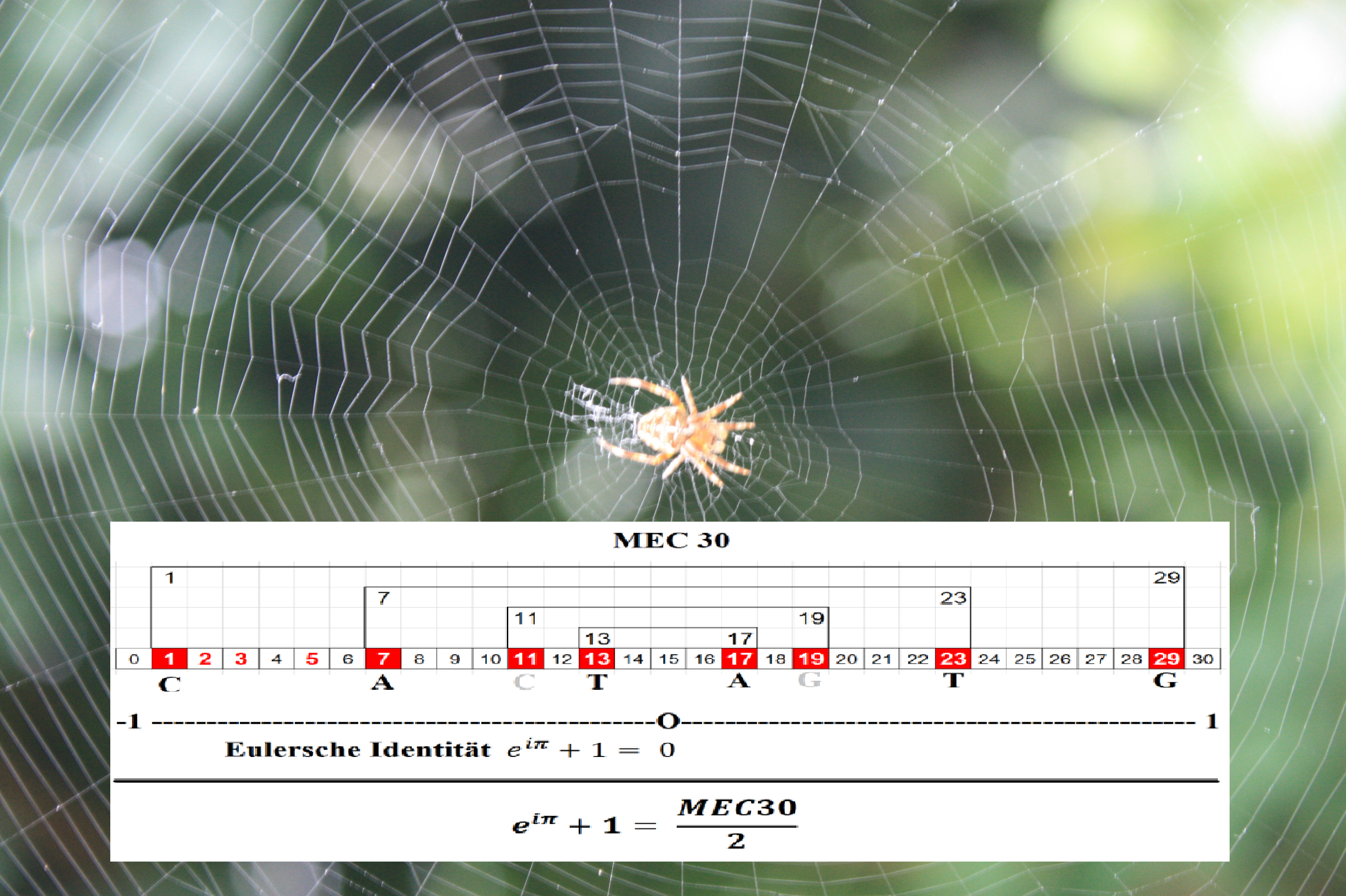The only different is, instead of an instance, it will behave as an inside container, just like how spider built a home web as strong as steel but useless to cover them against a rainy day nor even a small breeze.